# 3x square

The side length of the square is 54 cm. How many times increases the content area of a square if the length of side increase three times?

n =  9

### Step-by-step explanation:

$n={3}^{2}=9$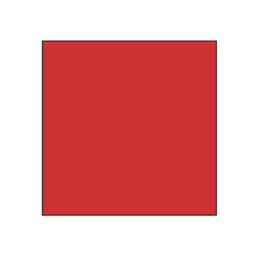Did you find an error or inaccuracy? Feel free to write us. Thank you!Tips to related online calculators
Do you want to convert length units?

#### You need to know the following knowledge to solve this word math problem:

We encourage you to watch this tutorial video on this math problem:

## Related math problems and questions:

• Tripled squareIf you tripled the length of the sides of the square ABCD you increases its content by 200 cm2. How long is the side of the square ABCD?
• Trapezium zoomHow many times increase area of trapezoid if all sides and altitude increase 5 times?
• Square - increased perimeterHow many times is increased perimeter of the square, where its sides increases by 150%? If the perimeter of square will increase twice, how much% increases the content area of the square?
• SquareSuppose the square's sides' length decreases by a 25% decrease in the content area of 28 cm2. Determine the side length of the original square.
• Star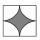Calculate the content of the shaded part of square with side 28 cm.
• Calculate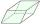Calculate the content cm2 diamond with side length 9.8 cm and a height on the side of 5 cm.
• The schoolyardThe schoolyard had the shape of a square with an 11m side. The yard has been enlarged by 75 m2 and has a square shape again. How many meters was each side of the yard enlarged?
• Rectangle vs squareOne side of the rectangle is 1 cm shorter than the side of the square, the second side is 3 cm longer than the side of the square. Square and rectangle have the same content. Calculate the length of the sides of a square and a rectangle.
• Square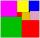If we increase one side of square by its one-half then square perimeter increase by 10 cm. What is the side of the square?
• Square and rectangleCalculate the side of a square which content area equals area of the rectangle having a length of 3 cm greater and by 2 cm smaller than the side of the square.
• Ratio - rectangleThe rectangle has dimensions 6 cm and 9 cm. How many times increases area and its perimeter, when its dimensions increase in the ratio 5:3?
• Magnification of the square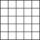If we increase the square side, increase the content of the 70 %. About what percentage was increased his sides?
• Square gridSquare grid consists of a square with sides of length 1 cm. Draw in it at least three different patterns such that each had a content of 6 cm2 and circumference 12 cm and that their sides is in square grid.
• CircleHow big is an area of a circle if its circumference is 51.2 cm?
• The cube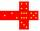The surface of the cube is 150 square centimeters. Calculate: a- the content of its walls b - the length of its edges
• Diagonals in the diamondThe length of one diagonal in diamond is 24 cm greater than the length of the second diagonal and diamond area is 50 m2. Determine the sizes of the diagonals.
• Extending square gardenMrs. Petrová's garden had the shape of a square with a side length of 15 m. After its enlargement by 64 m2 (square), it had the shape of a square again. How many meters has the length of each side of the garden been extended?